# Titrimetrey as analytical tool, P K MANI

20 de Jan de 2014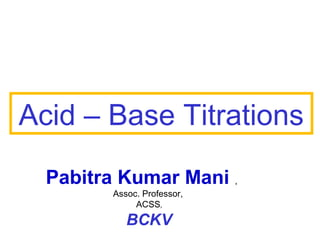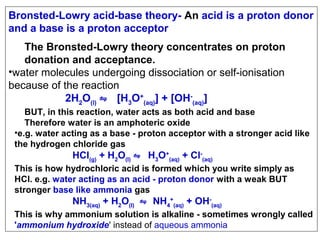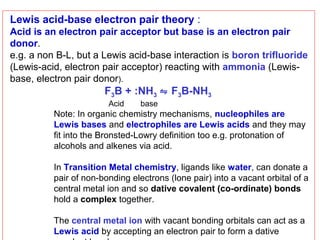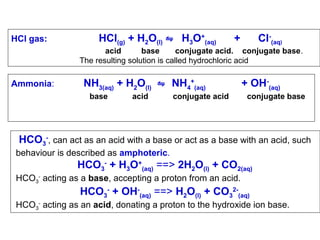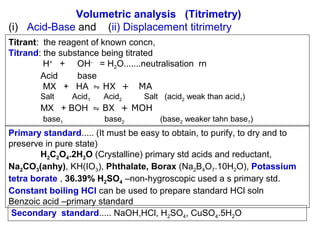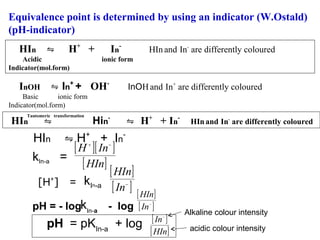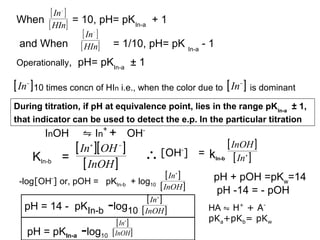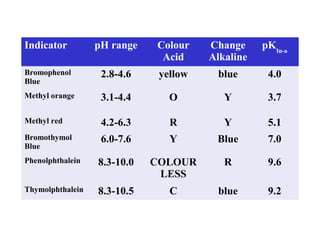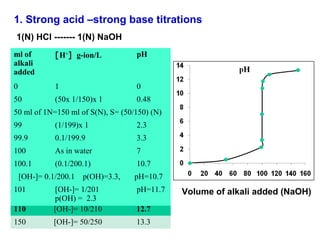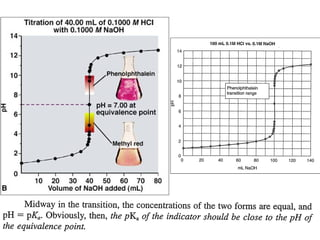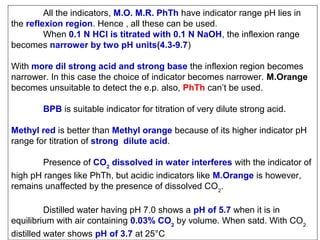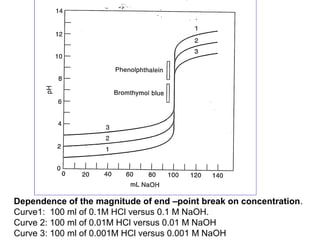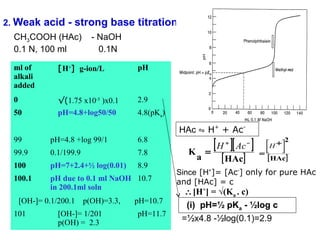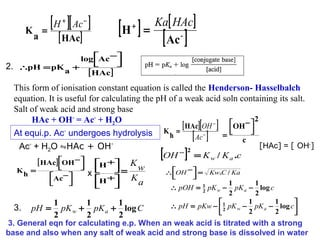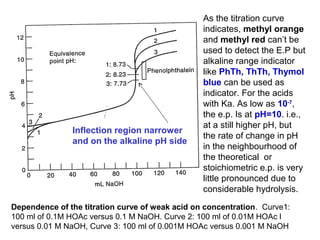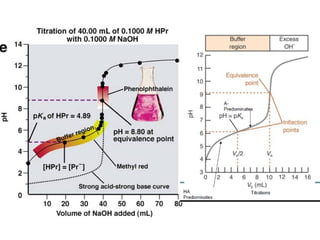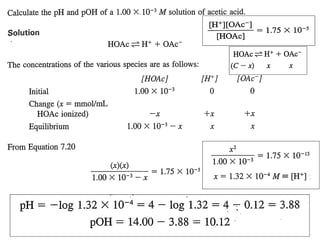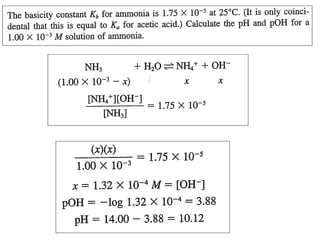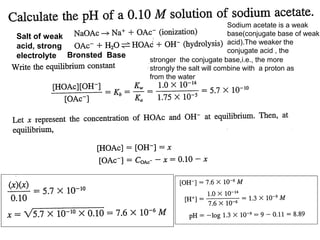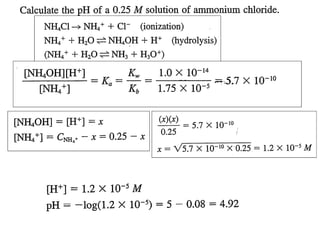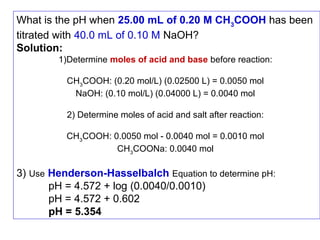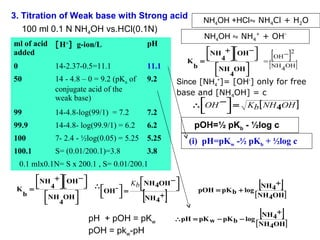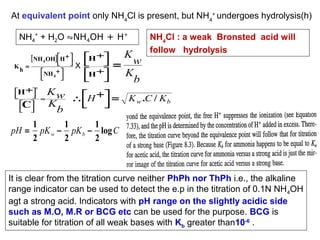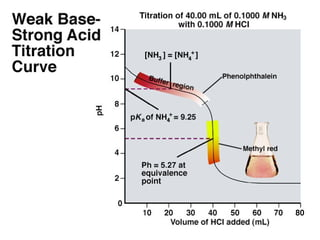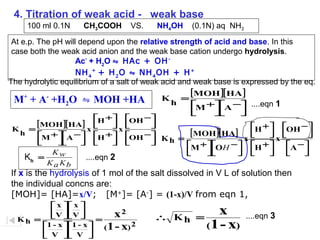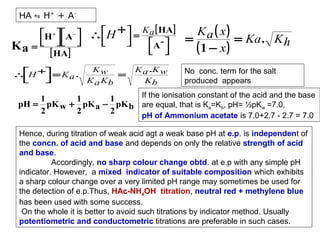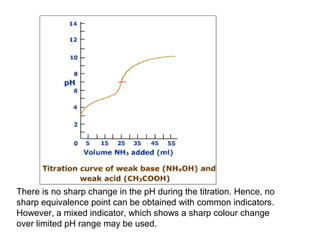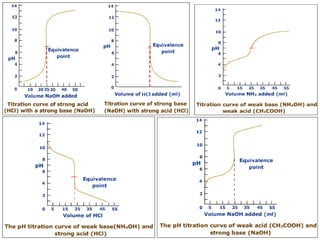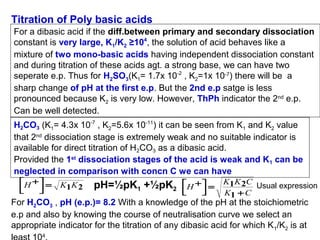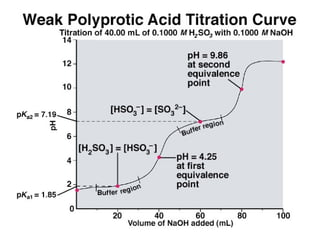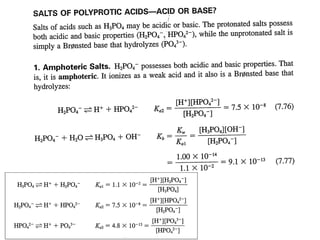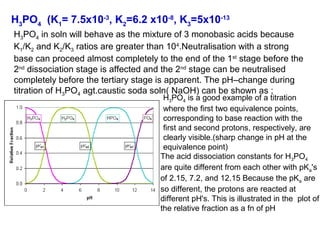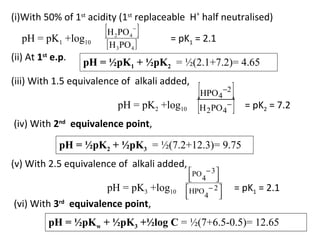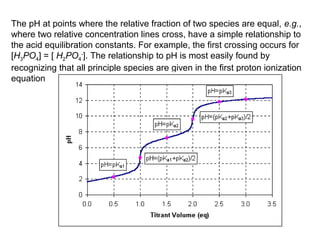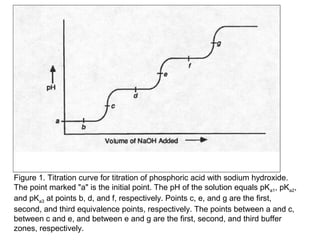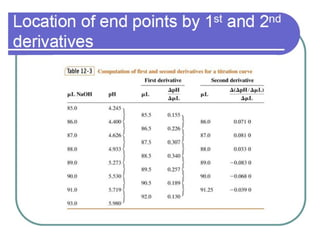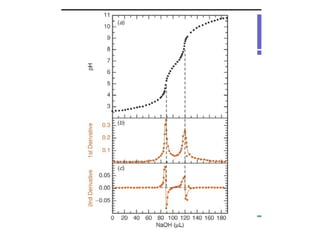1 de 37

### Titrimetrey as analytical tool, P K MANI

• 1. Acid – Base Titrations Pabitra Kumar Mani , Assoc. Professor, ACSS, BCKV
• 2. Bronsted-Lowry acid-base theory- An acid is a proton donor and a base is a proton acceptor The Bronsted-Lowry theory concentrates on proton donation and acceptance. •water molecules undergoing dissociation or self-ionisation because of the reaction 2H2O(l) ⇋ [H3O+(aq)] + [OH-(aq)] BUT, in this reaction, water acts as both acid and base Therefore water is an amphoteric oxide •e.g. water acting as a base - proton acceptor with a stronger acid like the hydrogen chloride gas HCl(g) + H2O(l) ⇋ H3O+(aq) + Cl-(aq) This is how hydrochloric acid is formed which you write simply as HCl. e.g. water acting as an acid - proton donor with a weak BUT stronger base like ammonia gas NH3(aq) + H2O(l) ⇋ NH4+(aq) + OH-(aq) This is why ammonium solution is alkaline - sometimes wrongly called 'ammonium hydroxide' instead of aqueous ammonia
• 3. Lewis acid-base electron pair theory : Acid is an electron pair acceptor but base is an electron pair donor. e.g. a non B-L, but a Lewis acid-base interaction is boron trifluoride (Lewis-acid, electron pair acceptor) reacting with ammonia (Lewisbase, electron pair donor). F3B + :NH3 ⇋ F3B-NH3 Acid base Note: In organic chemistry mechanisms, nucleophiles are Lewis bases and electrophiles are Lewis acids and they may fit into the Bronsted-Lowry definition too e.g. protonation of alcohols and alkenes via acid. In Transition Metal chemistry, ligands like water, can donate a pair of non-bonding electrons (lone pair) into a vacant orbital of a central metal ion and so dative covalent (co-ordinate) bonds hold a complex together. The central metal ion with vacant bonding orbitals can act as a Lewis acid by accepting an electron pair to form a dative
• 4. HCl gas: HCl(g) + H2O(l) ⇋ H3O+(aq) + Cl-(aq) acid base conjugate acid. conjugate base. The resulting solution is called hydrochloric acid Ammonia: NH3(aq) + H2O(l) ⇋ NH4+(aq) base acid conjugate acid + OH-(aq) conjugate base HCO3-, can act as an acid with a base or act as a base with an acid, such behaviour is described as amphoteric. HCO3- + H3O+(aq) ==> 2H2O(l) + CO2(aq) HCO3- acting as a base, accepting a proton from an acid. HCO3- + OH-(aq) ==> H2O(l) + CO32-(aq) HCO3- acting as an acid, donating a proton to the hydroxide ion base.
• 5. Volumetric analysis (Titrimetry) (i) Acid-Base and (ii) Displacement titrimetry Titrant: the reagent of known concn, Titrand: the substance being titrated H+ + OH- = H2O.......neutralisation rn Acid base MX + HA ⇋ HX + MA Salt Acid1 Acid2 Salt (acid2 weak than acid1) MX + BOH ⇋ BX + MOH base1 base2 (base2 weaker tahn base1) Primary standard..... (It must be easy to obtain, to purify, to dry and to preserve in pure state) H2C2O4.2H2O (Crystalline) primary std acids and reductant, Na2CO3(anhy), KH(IO3), Phthalate, Borax (Na2B4O7.10H2O), Potassium tetra borate , 36.39% H2SO4 –non-hygroscopic used a s primary std. Constant boiling HCl can be used to prepare standard HCl soln Benzoic acid –primary standard Secondary standard..... NaOH,HCl, H2SO4, CuSO4.5H2O
• 6. Equivalence point is determined by using an indicator (W.Ostald) (pH-indicator) HIn ⇋ H+ + Acidic Indicator(mol.form) InOH In- HIn and In- are differently coloured ionic form ⇋ In+ + OH- InOH and In+ are differently coloured Basic ionic form Indicator(mol.form) ⇋ Tautomeric transformation HIn Hin* ⇋ H+ + In- HIn and In- are differently coloured HIn ⇋ H+ + In[ H ][ In ] kIn-a = + [ HIn] [H ] + = kIn-a pH = - logkIn-a − [ HIn] [ In ] [ HIn] − - log [ In ] − pH = pKIn-a + log [ In ] − [ HIn] Alkaline colour intensity acidic colour intensity
• 7. [ In ] When [ HIn] = 10, pH= pKIn-a + 1 [ In ] and When [ HIn] = 1/10, pH= pK In-a - 1 − − Operationally, pH= pKIn-a ± 1 [ In ] 10 times concn of HIn i.e., when the color due to [ In ] is dominant − − During titration, if pH at equivalence point, lies in the range pK In-a ± 1, that indicator can be used to detect the e.p. In the particular titration InOH ⇋ In+ + OH- [ In ][ OH ] = [ InOH ] + KIn-b − [ InOH ] ∴ [OH ] = kIn-b [ In ] - [ In ] + -log[OH ] or, pOH = pKIn-b + log10 - [ InOH ] [ In ] pH = 14 - pKIn-b -log10 [ InOH ] + [ In ] + pH = pKIn-a -log10 [ InOH ] + pH + pOH =pKw=14 pH -14 = - pOH HA ⇋ H+ + ApKa+pKb= pKw
• 8. Indicator pH range Colour Acid Change Alkaline pKIn-a Bromophenol Blue 2.8-4.6 yellow blue 4.0 Methyl orange 3.1-4.4 O Y 3.7 Methyl red 4.2-6.3 R Y 5.1 Bromothymol Blue 6.0-7.6 Y Blue 7.0 Phenolphthalein 8.3-10.0 COLOUR LESS R 9.6 Thymolphthalein 8.3-10.5 C blue 9.2
• 9. 1. Strong acid –strong base titrations 1(N) HCl ------- 1(N) NaOH ml of alkali added [H+] g-ion/L pH 0 1 0 50 (50x 1/150)x 1 0.48 50 ml of 1N=150 ml of S(N), S= (50/150) (N) 99 (1/199)x 1 2.3 99.9 0.1/199.9 3.3 100 As in water 7 100.1 (0.1/200.1) 10.7 [OH-]= 0.1/200.1 101 p(OH)=3.3, pH=10.7 110 [OH-]= 1/201 p(OH) = 2.3 [OH-]= 10/210 pH=11.7 12.7 150 [OH-]= 50/250 13.3 Volume of alkali added (NaOH)
• 11. All the indicators, M.O. M.R. PhTh have indicator range pH lies in the reflexion region. Hence , all these can be used. When 0.1 N HCl is titrated with 0.1 N NaOH, the inflexion range becomes narrower by two pH units(4.3-9.7) With more dil strong acid and strong base the inflexion region becomes narrower. In this case the choice of indicator becomes narrower. M.Orange becomes unsuitable to detect the e.p. also, PhTh can’t be used. BPB is suitable indicator for titration of very dilute strong acid. Methyl red is better than Methyl orange because of its higher indicator pH range for titration of strong dilute acid. Presence of CO2 dissolved in water interferes with the indicator of high pH ranges like PhTh, but acidic indicators like M.Orange is however, remains unaffected by the presence of dissolved CO2. Distilled water having pH 7.0 shows a pH of 5.7 when it is in equilibrium with air containing 0.03% CO2 by volume. When satd. With CO2 distilled water shows pH of 3.7 at 25°C
• 12. Dependence of the magnitude of end –point break on concentration. Curve1: 100 ml of 0.1M HCl versus 0.1 M NaOH. Curve 2: 100 ml of 0.01M HCl versus 0.01 M NaOH Curve 3: 100 ml of 0.001M HCl versus 0.001 M NaOH
• 13. 2. Weak acid - strong base titration CH3COOH (HAc) 0.1 N, 100 ml - NaOH 0.1N ml of alkali added [H+] g-ion/L pH 0 √(1.75 x10-5 )x0.1 pH=4.8+log50/50 2.9 50 4.8(pKa) HAc ⇋ H+ + Ac99 pH=4.8 +log 99/1 6.8 99.9 0.1/199.9 7.8 100 pH=7+2.4+½ log(0.01) 8.9 100.1 pH due to 0.1 ml NaOH 10.7 in 200.1ml soln [OH-]= 0.1/200.1 101 p(OH)=3.3, [OH-]= 1/201 p(OH) = 2.3 pH=10.7 pH=11.7 [ H ][ Ac ] = + K a [ HAc] − =  + 2 H      [ HAc] Since [H+]= [Ac-] only for pure HAc and [HAc] = c ∴[H+] = √(Ka . c) (i) pH=½ pKa - ½log c =½x4.8 -½log(0.1)=2.9
• 14. [ H ][ Ac ] = + K a [ HAc] − [H ] + Ka[ HAc] = Ac - [ ] log Ac −     2. ∴pH = pK a + [ HAc] This form of ionisation constant equation is called the Henderson- Hasselbalch equation. It is useful for calculating the pH of a weak acid soln containing its salt. Salt of weak acid and strong base 2 HAc + OH- = Ac- + H2O − OH −  [ HAc] [ OH ]   K =  At equi.p. Ac undergoes hydrolysis − = h [ Ac ] c [HAc] = [ OH-] Ac- + H2O ⇋HAc + OH2 OH − = K w / K a .c [ HAc] OH −  H + K     w   K = ∴OH −  = Kw.C / Ka x  + = h −   Ac   K H    a   1 1   1   [ ] ∴ pOH = 2 pK w − 3. pH = 1 1 1 pK w + pK a + log C 2 2 2 pK a − log c 2 1 1   ∴ pH = pKw −  1 pK w − pK a − log c  2 2 2   2 3. General eqn for calculating e.p. When an weak acid is titrated with a strong base and also when any salt of weak acid and strong base is dissolved in water
• 15. Inflection region narrower and on the alkaline pH side As the titration curve indicates, methyl orange and methyl red can’t be used to detect the E.P but alkaline range indicator like PhTh, ThTh, Thymol blue can be used as indicator. For the acids with Ka. As low as 10-7, the e.p. Is at pH=10. i.e., at a still higher pH, but the rate of change in pH in the neighbourhood of the theoretical or stoichiometric e.p. is very little pronounced due to considerable hydrolysis. Dependence of the titration curve of weak acid on concentration. Curve1: 100 ml of 0.1M HOAc versus 0.1 M NaOH. Curve 2: 100 ml of 0.01M HOAc l versus 0.01 M NaOH, Curve 3: 100 ml of 0.001M HOAc versus 0.001 M NaOH
• 19. Salt of weak acid, strong electrolyte Bronsted Base Sodium acetate is a weak base(conjugate base of weak acid).The weaker the conjugate acid , the stronger the conjugate base,i.e., the more strongly the salt will combine with a proton as from the water
• 21. What is the pH when 25.00 mL of 0.20 M CH3COOH has been titrated with 40.0 mL of 0.10 M NaOH? Solution: 1)Determine moles of acid and base before reaction: CH3COOH: (0.20 mol/L) (0.02500 L) = 0.0050 mol NaOH: (0.10 mol/L) (0.04000 L) = 0.0040 mol 2) Determine moles of acid and salt after reaction: CH3COOH: 0.0050 mol - 0.0040 mol = 0.0010 mol CH3COONa: 0.0040 mol 3) Use Henderson-Hasselbalch Equation to determine pH: pH = 4.572 + log (0.0040/0.0010) pH = 4.572 + 0.602 pH = 5.354
• 22. 3. Titration of Weak base with Strong acid 100 ml 0.1 N NH4OH vs.HCl(0.1N) ml of acid [H+] g-ion/L added pH 0 14-2.37-0.5=11.1 11.1 50 14 - 4.8 – 0 = 9.2 (pKa of conjugate acid of the weak base) 9.2 99 14-4.8-log(99/1) = 7.2 7.2 99.9 14-4.8- log(99.9/1) = 6.2 6.2 100 7- 2.4 - ½log(0.05) = 5.25 5.25 100.1 S= (0.01/200.1)=3.8 3.8 NH4OH +HCl⇋ NH4Cl + H2O NH4OH ⇋ NH4+ + OH- [ Since [NH4+]= [OH-] only for free base and [NH4OH] = c ∴OH −  = Kb [ NH 4OH ]     pOH=½ pKb - ½log c (i) pH=pKw -½ pKb + ½log c 0.1 mlx0.1N= S x 200.1 , S= 0.01/200.1 NH +  OH −      4  K = b NH OH      4 Kb NH 4OH −      OH -  =     NH 4+ ∴ [ pH + pOH = pKw pOH = pkw-pH ] NH +  OH −  −2    = OH   4  K = [NH 4OH] b NH OH      4 ] pOH = pK b + log ∴pH = pK w − pK b − log [NH4+ ] [ NH4OH ] [NH4+ ] [ NH4OH ]
• 23. At equivalent point only NH4Cl is present, but NH4+ undergoes hydrolysis(h) NH4+ + H2O ⇋NH4OH + H+ K h = H +           C H +    X     NH +  H + 4         [ NH 4 OH ] H +    2 = = K w K b NH4Cl : a weak Bronsted acid will follow hydrolysis Kw ∴H +  = K w .C / K b   K   b 1 1 1 pH = pK w − pK b − log C 2 2 2 It is clear from the titration curve neither PhPh nor ThPh i.e., the alkaline range indicator can be used to detect the e.p in the titration of 0.1N NH 4OH agt a strong acid. Indicators with pH range on the slightly acidic side such as M.O, M.R or BCG etc can be used for the purpose. BCG is suitable for titration of all weak bases with Kb greater than10-6 .
• 25. 4. Titration of weak acid - weak base 100 ml 0.1N CH3COOH VS. NH4OH (0.1N) aq NH3 At e.p. The pH will depend upon the relative strength of acid and base. In this case both the weak acid anion and the weak base cation undergo hydrolysis. Ac- + H2O ⇋ HAc + OH NH 4 + + H 2 O ⇋ NH 4 OH + H + The hydrolytic equilibrium of a salt of weak acid and weak base is expressed by the eq. [ΜΟΗ ][ΗΑ ] + Κ = M + A +H2O ⇋ MOH +HA h Μ +Α − ....eqn 1       H +  [ΜΟΗ ][ΗΑ ] x   x   Κ = h Μ + Α − H +            Kw Kh = ....eqn 2 K a Kb OH −     [ ΜΟΗ ][ ΗΑ ] x OH − Κh =     Μ + OH −        H +  OH −          x H +  A −          If x is the hydrolysis of 1 mol of the salt dissolved in V L of solution then the individual concns are: [MOH]= [HA]=x/V; [M+]= [A-] = (1-x)/V from eqn 1,  x  x  V V  x2    = Κh = 1 - x 1 - x  (1 - x) 2  V  V     ∴ Kh = x (1 - x) ....eqn 3
• 26. HA ⇋ H+ + A- Ka H +   A −     =    [ HA ]  ∴ H + = K a .     ∴ H +  = K a [ HA ] = K a ( x ) = Ka . K   A -  h   (1 − x)     Kw = K a Kb K a .K w Kb No conc. term for the salt produced appears If the ionisation constant of the acid and the base 1 1 1 pH = pK w + pK a − pK b are equal, that is Ka=Kb, pH= ½pKw =7.0, 2 2 2 pH of Ammonium acetate is 7.0+2.7 - 2.7 = 7.0 Hence, during titration of weak acid agt a weak base pH at e.p. is independent of the concn. of acid and base and depends on only the relative strength of acid and base. Accordingly, no sharp colour change obtd. at e.p with any simple pH indicator. However, a mixed indicator of suitable composition which exhibits a sharp colour change over a very limited pH range may sometimes be used for the detection of e.p.Thus, HAc-NH4OH titration, neutral red + methylene blue has been used with some success. On the whole it is better to avoid such titrations by indicator method. Usually potentiometric and conductometric titrations are preferable in such cases.
• 27. There is no sharp change in the pH during the titration. Hence, no sharp equivalence point can be obtained with common indicators. However, a mixed indicator, which shows a sharp colour change over limited pH range may be used.
• 29. Titration of Poly basic acids For a dibasic acid if the diff.between primary and secondary dissociation constant is very large, K1/K2 ≥104, the solution of acid behaves like a mixture of two mono-basic acids having independent dissociation constant and during titration of these acids agt. a strong base, we can have two seperate e.p. Thus for H2SO3(K1= 1.7x 10-2 , K2=1x 10-7) there will be a sharp change of pH at the first e.p. But the 2nd e.p satge is less pronounced because K2 is very low. However, ThPh indicator the 2nd e.p. Can be well detected. H2CO3 (K1= 4.3x 10-7 , K2=5.6x 10-11) it can be seen from K1 and K2 value that 2nd dissociation stage is extremely weak and no suitable indicator is available for direct titration of H2CO3 as a dibasic acid. Provided the 1st dissociation stages of the acid is weak and K1 can be neglected in comparison with concn C we can have H +  =     K1K 2 pH=½pK1 +½pK2 H +  =     K1K 2C Usual expression K1 + C For H2CO3 , pH (e.p.)= 8.2 With a knowledge of the pH at the stoichiometric e.p and also by knowing the course of neutralisation curve we select an appropriate indicator for the titration of any dibasic acid for which K 1/K2 is at 4
• 32. H3PO4 (K1= 7.5x10-3, K2=6.2 x10-8, K3=5x10-13 H3PO4 in soln will behave as the mixture of 3 monobasic acids because K1/K2 and K2/K3 ratios are greater than 104.Neutralisation with a strong base can proceed almost completely to the end of the 1st stage before the 2nd dissociation stage is affected and the 2nd stage can be neutralised completely before the tertiary stage is apparent. The pH–change during titration of H3PO4 agt.caustic soda soln( NaOH) can be shown as ; H3PO4 is a good example of a titration where the first two equivalence points, corresponding to base reaction with the first and second protons, respectively, are clearly visible.(sharp change in pH at the equivalence point) The acid dissociation constants for H3PO4 are quite different from each other with pKa's of 2.15, 7.2, and 12.15 Because the pKa are so different, the protons are reacted at different pH's. This is illustrated in the plot of the relative fraction as a fn of pH
• 33. (i)With 50% of 1st acidity (1st replaceable H+ half neutralised) [H PO ] − pH = pK1 +log10 (ii) At 1st e.p. 2 4 [ H 3PO 4 ] = pK1 = 2.1 pH = ½pK1 + ½pK2 = ½(2.1+7.2)= 4.65 (iii) With 1.5 equivalence of alkali added, pH = pK2 +log10 [HPO ] [H PO ] 4 2 −2 4 − = pK2 = 7.2 (iv) With 2nd equivalence point, pH = ½pK2 + ½pK3 = ½(7.2+12.3)= 9.75 (v) With 2.5 equivalence of alkali added, pH = pK3 +log10 (vi) With 3rd equivalence point, PO − 3   4    HPO − 2   4    = pK1 = 2.1 pH = ½pKw + ½pK3 +½log C = ½(7+6.5-0.5)= 12.65
• 34. The pH at points where the relative fraction of two species are equal, e.g., where two relative concentration lines cross, have a simple relationship to the acid equilibration constants. For example, the first crossing occurs for [H3PO4] = [ H2PO4-]. The relationship to pH is most easily found by recognizing that all principle species are given in the first proton ionization equation
• 35. Figure 1. Titration curve for titration of phosphoric acid with sodium hydroxide. The point marked "a" is the initial point. The pH of the solution equals pKa1, pKa2, and pKa3 at points b, d, and f, respectively. Points c, e, and g are the first, second, and third equivalence points, respectively. The points between a and c, between c and e, and between e and g are the first, second, and third buffer zones, respectively.